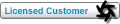## Curves and Cd in Octane 2018 not working Tweet

Houdini Integrated Plugin

Moderator: juanjgon

### Re: Curves and Cd in Octane 2018 not working

i forgot to post the code you need to put inside a Primitive Wrangle to export @Cd and @Alpha by using @uv.
you are able to export them inside your .abc. and use rgb and alpha .ppm map directly.

Code: Select all
`int base       = 64;int resolution = 4096;float r = @Cd.x;float g = @Cd.y;float b = @Cd.z;float a = @Alpha;float min = 0.04;float max = 0.96;if(r<min) r = min; if(r>max) r = max;if(g<min) g = min; if(g>max) g = max;if(b<min) b = min; if(b>max) b = max;if(a<min) a = min; if(a>max) a = max;int rl = r*(base-1);int gl = g*(base-1);int bl = b*(base-1);int al = a*(base-1); int rgbLine = al + bl*base + gl*base*base + rl*base*base*base; int xPix    = rgbLine%resolution;int yPix    = rgbLine/resolution;   [email protected] = (float)xPix/resolution;[email protected] = 1.0 - (float)yPix/resolution;`

it work for curves in standalone
it doesn't work on point due to abc importer restrictionfantomePosts: 132
Joined: Wed Dec 16, 2015 3:38 pm
Previous